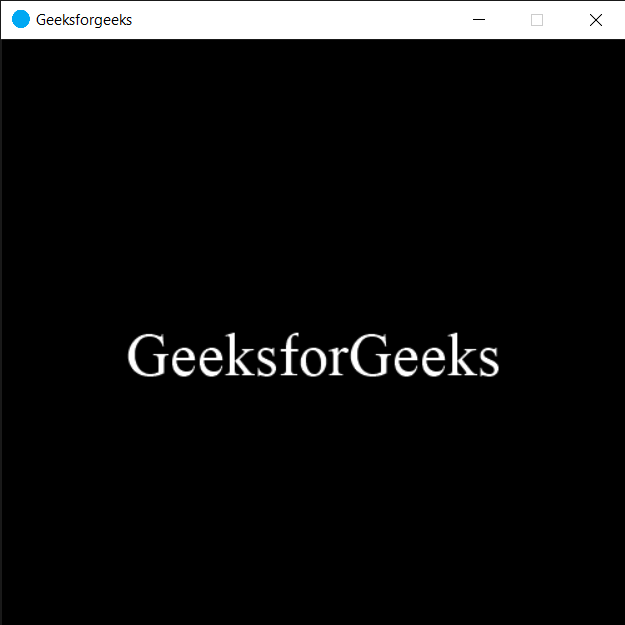Open in App
Not now

# PYGLET – On Move Event

• Last Updated : 08 Oct, 2021

In this article we will see how we can trigger on move event in PYGLET module in python. Pyglet is easy to use but powerful library for developing visually rich GUI applications like games, multimedia etc. A window is a “heavyweight” object occupying operating system resources. Windows may appear as floating regions or can be set to fill an entire screen (fullscreen).This event get called when the window get moved, we can change the position of the window with the help of set_location method.

We can create a window with the help of command given below

`pyglet.window.Window(width, height, title)`

Below is the syntax of the on move event, this method get called when this event is triggered

```@window.event
def on_move(x, y):
print("Window Moved")```

Below is the implementation

## Python3

 `# importing pyglet module``import` `pyglet``import` `pyglet.window.key` `# width of window``width ``=` `500` `# height of window``height ``=` `500` `# caption i.e title of the window``title ``=` `"Geeksforgeeks"` `# creating a window``window ``=` `pyglet.window.Window(width, height, title)` `# text``text ``=` `"GeeksforGeeks"` `# creating a label with font = times roman``# font size = 36``# aligning it to the center``label ``=` `pyglet.text.Label(text,``                          ``font_name ``=``'Times New Roman'``,``                          ``font_size ``=` `36``,``                          ``x ``=` `window.width``/``/``2``, y ``=` `window.height``/``/``2``,``                          ``anchor_x ``=``'center'``, anchor_y ``=``'center'``)` `new_label ``=` `pyglet.text.Label(text,``                          ``font_name ``=``'Times New Roman'``,``                          ``font_size ``=` `10``,``                          ``x ``=` `25``, y ``=` `25``)` `# on draw event``@window``.event``def` `on_draw():   ` `    ` `    ``# clearing the window``    ``window.clear()``    ` `    ``# drawing the label on the window``    ``label.draw()` `    ` `# key press event   ``@window``.event``def` `on_key_press(symbol, modifier):` `    ``# key "C" get press``    ``if` `symbol ``=``=` `pyglet.window.key.C:``        ` `        ``print``(``"Key C is pressed"``)`  `# on move event``@window``.event``def` `on_move(x, y):``    ` `    ``# printing some message``    ``print``(``"Window Move"``)``    ``print``(``"New Position : "``, end ``=` `"")``    ``print``(x, y)``    `  ` `  `# image for icon``img ``=` `image ``=` `pyglet.resource.image(``"logo.png"``)` `# setting image as icon``window.set_icon(img)` `# moving window``window.set_location(``100``, ``200``)``               ` `# start running the application``pyglet.app.run()`

Output :```Window Move
New Position : 100 200```

My Personal Notes arrow_drop_up